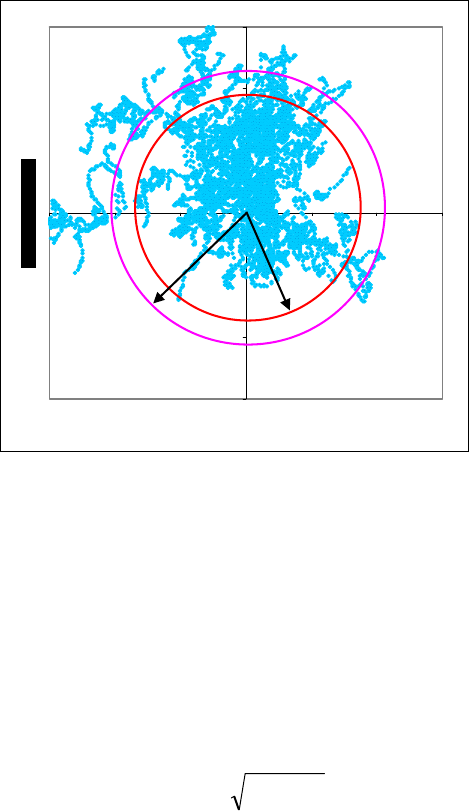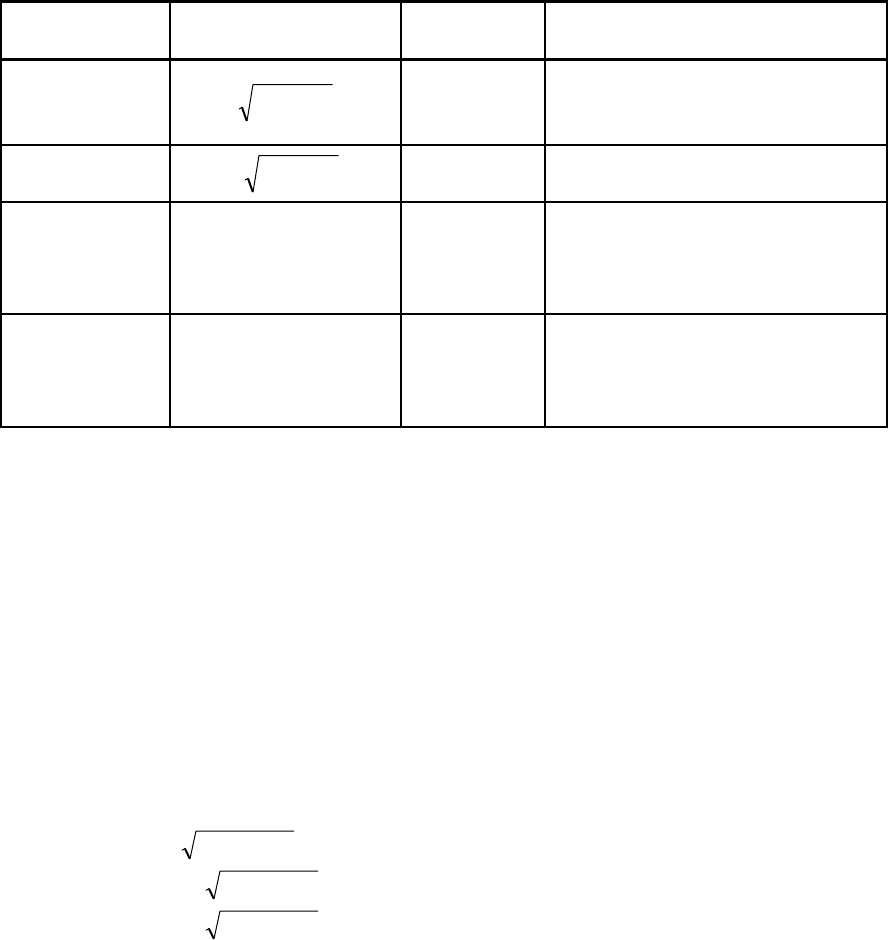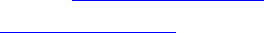APN-029 Rev 1 December 03, 2003
1 of 6
GPS Position Accuracy Measures
Overview
There are various statistical methods of describing specifications for GPS receiver and it
can seem confusing or misleading the meaning of such statistics. The purpose of this
document is to introduce some of the most commonly used accuracy measures, and their
relationships to one another.
1 Accuracy and Precision
Accuracy and precision are often used to describe the how good is the position acquired
by GPS receiver. A distinction should be made between accuracy and precision.
Accuracy is the degree of closeness of an estimate to its true, but unknown value and the
precision is the degree of closeness of observations to their means. Figure 1 illustrates
various relationships between these two parameters. The true value is located at the
intersection of the crosshairs, the center of the shaded area is the location of the mean
estimate, and the radius of the shaded area is a measure of the uncertainty contained in
the estimate.
Figure 1: Accuracy versus Precision
High accuracy,
Low precision
Low accuracy,
High precision
High accuracy,
High precision
Low accuracy,
Low precision2 of 6
2 Accuracy Measures - 2D
When GPS positions are logged over time, the positions are scattered over an area due to
measurement errors. This dispersion of points is called a scatter plot, which GPS
manufacturers use to characterize their equipment’s accuracy. The area within which the
measurements or estimated parameters are likely to be is called the confidence region.
The confidence region is then analyzed to quantify the GPS performance statistically.
The confidence region with a radius describes the probability that the solution will be
within the specified accuracy.
Figure 2 shows the scatter plot of the L1 single point positions observed by the actual
NovAtel OEM4-G2 receiver for 24 hours and its accuracy radii of CEP and DRMS.
-3
-2
-1
0
1
2
3
-3 -2 -1 0 1 2 3
East Error (m)
Figure 2: L1 Single Point positions collected on the rooftop of NovAtel building for 24
hours using NovAtel OEM4-G2 receiver and GPSAntenna Model 502.
2.1 Distance Root Mean Squared (DRMS)
DRMS is a single number that expresses 2D accuracy. In order to compute the DRMS of
horizontal position errors, the standard errors (σ) from the known position in the
directions of the coordinate axis are required.
DRMS is the square root of the average of the square errors which is defined as follows:
DRMS =
22
yx
σσ
+
! Standard errors (σ) of estimated coordinates (x, y) of each point being positioned
can be predicted from the corresponding variances on the diagonal of the
covariance matrix.
CEP
1.80 m
DRMS
2.16 m3 of 6
2.2 Circular Error Probability (CEP)
CEP refers to the radius of a circle in which 50% of the values occur, i.e. if a CEP of 5
meters is quoted then 50% of horizontal point positions should be within 5 meters of the
true position. The radius of the 95% is often quoted and the term R95 used. R95 is CEP
with the radius of the 95% probability circle.
Table 1 describes the most commonly used position accuracy measures and their
probability.
Table 1: 2D Position Accuracy Measures
Accuracy
Measures
Formula Probability Definition
DRMS
22
yx
σσ
+
65%
The square root of the average of
the squared horizontal position
errors.
2DRMS
2
22
yx
σσ
+
95%
Twice the DRMS of the
horizontal position errors.
CEP
xy
σ
σ
56.062.0 +
(Accurate when
3.0/ >
xy
σ
σ
)
50%
The radius of circle centered at
the true position, containing the
position estimate with
probability of 50%.
R95
R(
xy
σ
σ
56.062.0 + )
(R=2.08, when
1/ =
xy
σ
σ
)
95%
The radius of circle centered at
the true position, containing the
position estimate with
probability of 95%.
2.3 Examples of 2D Accuracy Measures
Example 1:
Given standard deviations:
σ
x
= 1.3 m
σ
y
= 1.5 m
Then accuracy measures can be calculated as:
CEP =
5.156.03.162.0 ×+× = 1.65 m
DRMS =
22
5.13.1 + = 1.98 m
2DRMS = 2
22
5.13.1 + = 3.96 m
3DRMS = 3
22
5.13.1 + = 5.85 m4 of 6
Example 2:
How to convert one accuracy measure to another?
When 1.8 meter of CEP is given, what is the position accuracy for 2DRMS?
Use Table 2 to find equivalent multiplier for the conversion and follow the steps below.
1. Go down the “2DRMS” column to the “CEP” row.
2. The multiplier in this cell is 2.4
3. CEP = 1.8 x 2.4 = 4.32 m
Example 3:
Which is more accurate, 1.8 meters of CEP or 3 meters of RMS (3D)?
1. In Table 4, go down the “RMS (3D)” to the “CEP” row.
2. The multiplier in this cell is 2.5
3. RMS (3D) = CEP x 2.5
4. CEP = RMS (3D) / 2.5 = 3 / 2.5 = 1.2
The position accuracy with 3 meters of RMS (3D) will be 1.25 meters of CEP, therefore
3 meters of RMS (3D) is more accurate than 1.8 meters of CEP.
Table 2 gives the multipliers required to go from one accuracy measure to another
assuming that σ
x
/ σ
y
= 1 and that VDOP / HDOP = 1.9 and PDOP / HDOP = 2.1.
Table 2: Equivalent Accuracy Multipliers
RMS
(Vertical)
CEP
RMS
(Horizontal)
R95
(Horizontal)
2DRMS
RMS
(3D)
SEP
1
0.44 0.53 0.91 1.1 1.1 0.88
RMS
(Vertical)
1
1.2 2.1 2.4 2.5 2.0
CEP
1
1.7 2 2.1 1.7
RMS
(Horizontal)
1
1.2 1.2 0.96
R95
1
1.1 0.85
2DRMS
1
0.79
RMS (3D)
1
SEP5 of 6
3 Accuracy Measures – 3D
Similar to 2D accuracy measures there are many representations of 3D accuracy with
various probabilities. 3D accuracy measures are conceptually similar to those in 2D
expanded by one dimension, the vertical accuracy. Spherical Error Probable (SEP)
corresponds to CEP in 2D, while Mean Radial Spherical Error (MRSE) corresponds to
DRMS in 2D.
Table 3 lists various methods for position accuracy measures in 3D and their definitions.
Table 3: 3D Position Accuracy Measures
Accuracy
Measures
Formula Probability Definition
SEP
(Spherical Error
Probable)
0.51(
zxy
σ
σ
σ
+
+ )
50%
centered at the true position,
containing the position
estimate in 3D with
probability of 50%
MRSE
Spherical Error)
222
zyx
σσσ
++
61%
centered at the true position,
containing the position
estimate in 3D with
probability of 61%
90% Spherical
Accuracy
Standard
0.833(
zxy
σ
σ
σ
+
+
)
90%
centered at the true position,
containing the position
estimate in 3D with
probability of 90%
99% Spherical
Accuracy
Standard
1.122(
zxy
σ
σ
σ
+
+
)
99%
centered at the true position,
containing the position
estimate in 3D with
probability of 99%
4 Dilution of Precision (DOP)
The DOPs provide a simple characterization of the user-satellite geometry. DOP is
related to the volume formed by the intersection points of the user-satellite vectors, with
the unit sphere centered on user. Larger volumes give smaller DOPs. Lower DOP values
generally represent better position accuracy. The role of DOP in GPS positioning,
however, is often misunderstood. A lower DOP value does not automatically mean a low
position error. The quality of a GPS-derived position estimate depends upon both the
measurement geometry as represented by DOP values, and range errors caused by signal
strength, ionospheric effects, multipath etc.6 of 6
5 Final Points
If you require any further information regarding the topics covered within this